Download PDF文章快速检索 高级检索

An algorithm for concept lattice construction based on maximum cycles of weight values
MAO Hua, LIU YichaoSchool of Mathematics and Information Science, Hebei University, Baoding 071002, China
Abstract: As an effective tool for knowledge discovery and data processing, the concept lattice has been widely applied in many fields. Searching all concepts in a formal context is a basic problem for research into concept lattice theory. On the basis of attribute topology and combined with the idea of graph theory, an algorithm to construct a concept lattice in a fixed formal context is given. The process is as follows:firstly, a weakened attribute topology was built up; then, by applying the method of searching the maximum cycle with a weight in the above weakened attribute topology, all of the formal context concepts were obtained; finally the concept lattice was established. Subsequent analysis illustrated that the algorithm can reduce complexity compared with some existing algorithms. In addition, using an example, the accuracy and validity of the algorithm was verified. The result presents a useful idea and method for knowledge acquisition.
Key words: formal context     concept lattice     concept     weight value     maximum cycle     attributes topology     data processing

1 基本概念

1.1 概念格

1)形式背景(O,M,I)是一个三元组，其中O是对象集，M是属性集，I⊆O×MOM中的元素分别称为对象和属性。

2)设A⊆OB⊆M,定义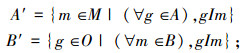A′=BB′=A，则元素对(A,B)是一个概念。A为概念(A,B)的外延，B为概念(A,B)的内涵。形式背景(O，M，I)的所有概念的集合用β(O,M,I)表示，称β(O,M,I)为(O,M,I)的概念格。

3)对于β(O,M,I)中的概念(A1,B1)和(A2,B2)，如果A1A2，我们写作(A1,B1)≤(A2,B2)。很容易看到(β(O,M,I)；≤)是一个完备格。

 对像 a b c d e f g 1 × × × 2 × × × × 3 × × × 4 × × × × 5 × × 6 ×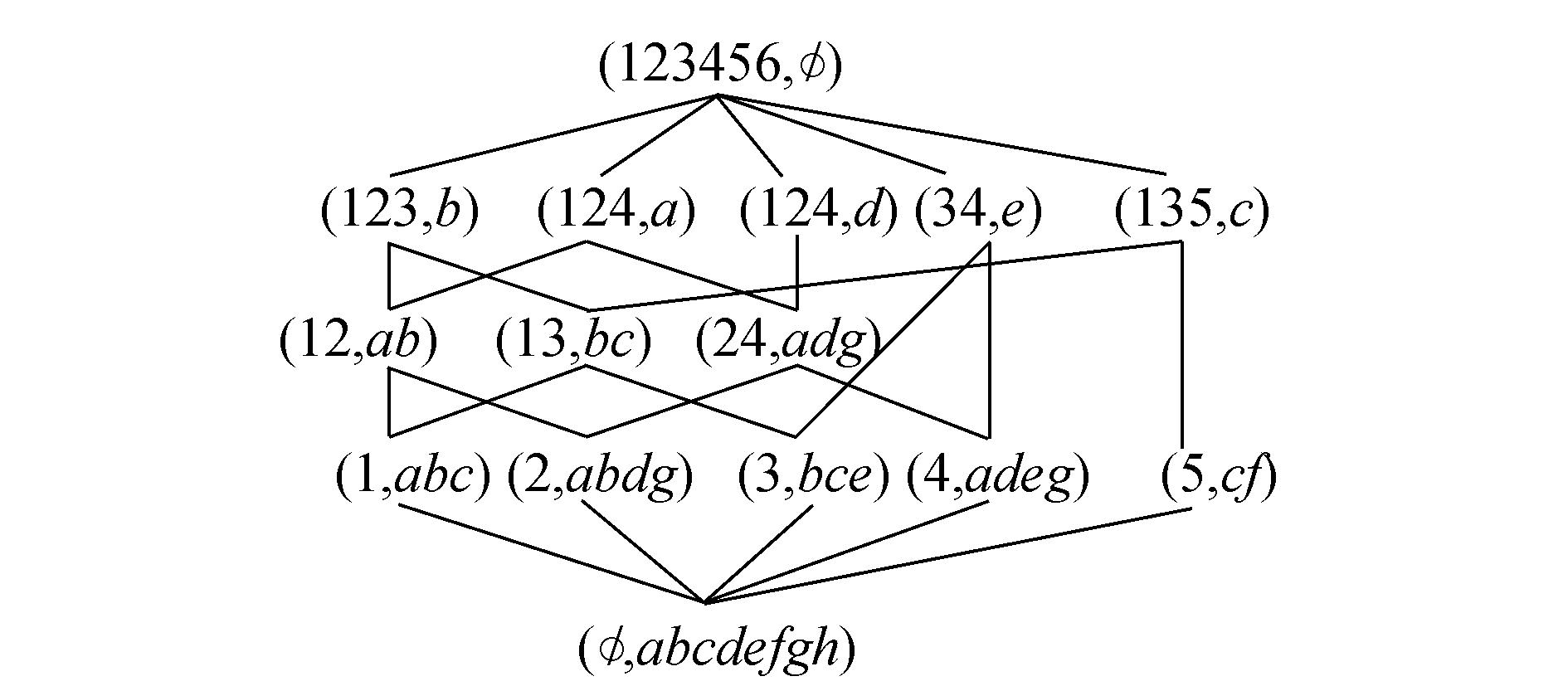图 1 β(O,M,I) Fig. 1 β(O,M,I)

1.2 图论

2)在顶边交错链P=v0e1v1e2vkek中，eiE(G)，i=1,2,…,kvjV(G)，j=1,2,…,k，且ei= vi-1 vi，则称PG的一条道路，其中允许vi= vjei= eji≠ j。称v0p的起点，vkp的终点。各顶相异的道路称为轨道；起点与终点重合的轨道称为圈。

3)在一个无向图中，只有一个顶的圈叫做自环；ψG(e1)= ψG(e2)=(u,v)，则称e1e2是重边。

1.3 属性拓扑图

1) 设m1m2Mm1m2

①若m′1m′2m′2m′1m′1m′2≠Ø，则用“↔”连接m1m2;

②若m′1m′2m′1m′2≠Ø，则用“→” 连接m1m2表示为m2m1;

③若m′1m′2 =Ø,则m1m2没有边连接。

2) 设(A(O,M,I)，w)为(O,M,I)的属性拓扑图，e(mi,mj)∈E(A(O,M,I)，w))，E(A(O,M,I)，w)为(A(O,M,I)，w)的边集，e(mi,mj)上的权值用w(mi,mj)表示，w(mi,mj)为属性mimj之间的公共对象{g1,g2,…,gn}的集合，称w(mi,mj)为mimj之间的权值。

3)设m∈Mb∈M，若与m连接的边均为非单向入边，即与m连接的边均为m→bm↔b，则称m为顶层属性，顶层属性的集合用T表示。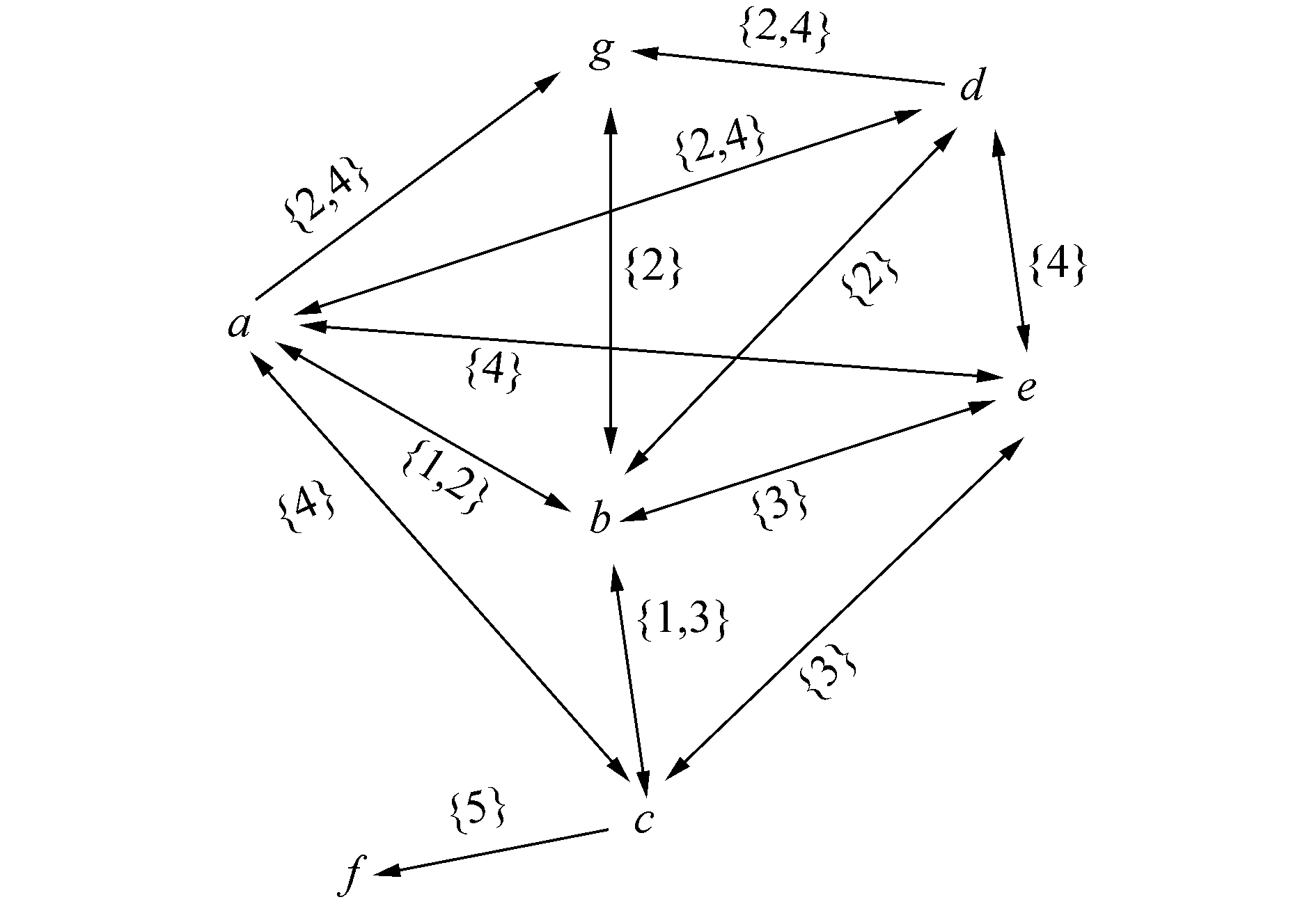图 2 (A(O,M,I)，w) Fig. 2 (A(O,M,I)，w)

2 概念格的构造

2.1 弱化的属性拓扑图

1)去掉属性拓扑图中的方向，得一无向图。

2)若m 在(A(O,M,I)，w)中为顶层属性，则在1)中的无向图中，加一个以m为顶点的自环。

3)若在1)中的无向图中，包含权值w(u,v)的只有一条边e，其中，u、ve的两个端点，则在uv之间再添加一条边e1 (图中用虚线表示)，并且令e1的权值也为w(u,v)。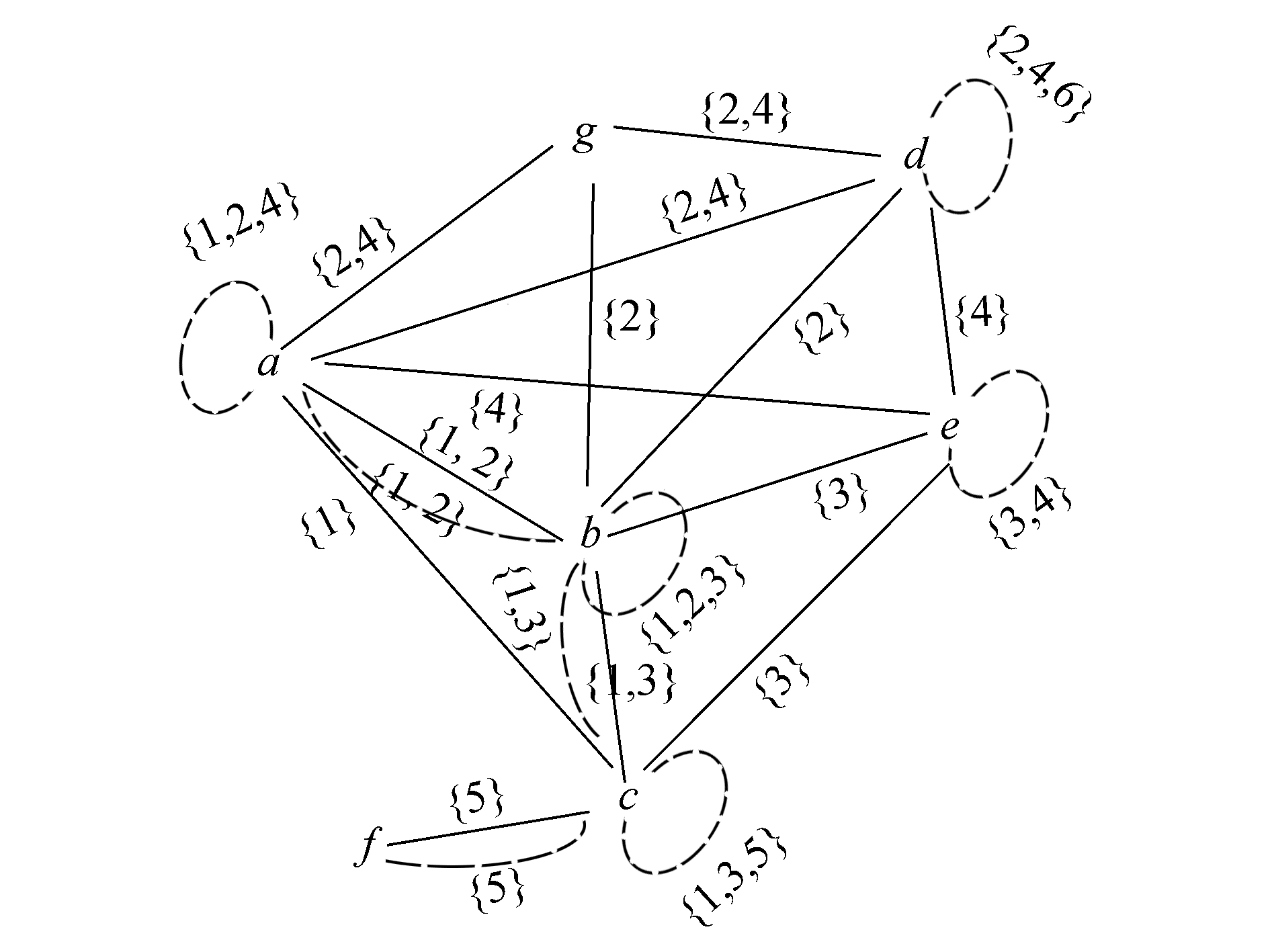图 3 (R(O,M,I)，w) Fig. 3 (R(O,M,I)，w)

2.2 算法过程

1)对于(O,M,I)，绘制属性拓扑图，根据属性拓扑图中的箭头指向，确定顶层属性集合T

2)将属性拓扑图转化为弱化的属性拓扑图。

3)①初始将W(R(O,M,I)，w)赋值给Wr，对任意wiwjWr，求wiwj, i,j=1,2,…,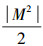。若wiwj=Ø或wiwj=wt，此处wi,wj,wtW(R(O,M,I)，w),i,j,t=1,2,…,。则进行4)。

②若wiwj=wtwtW(R(O,M,I)，w)，i,j,t=1,2,…,，则将Wrr={wtwiwj=wtwi,wj,∈W(R(O,M,I)，w)，wtW(R(O,M,I)，w)}添加到W(R(O,M,I)，w)中。将Wrr赋值给Wr，执行①。

4)取max|ws|，开始寻找边上权值包含ws的最大圈，记录权值ws最大圈的顶点为Y，对应概念为C={(A，B): A=ws ,B= Y}。

5)①根据4)的原则，若W中存在ws+1s=1,2,…,|M|2，则选定ws+1，重复4)。

②若W中不存在ws+1，则停止。

W(R(O,M,I)，w)中的元素满足3)中的①，若wiwj=Ø，或wiwj=wt，此处wi,wj,wtW(R(O,M,I)，w),i,j,t=1,2,…,，则能够进行4)、5)，又因为|W(R(O,M,I)，w)|是有限的，因此有限步后算法可以停止。

W(R(O,M,I)，w)中的元素满足wiwj=wt，wtW(R(O,M,I)，w)，i,j,t=1,2,…,，此时会将新生成的wt添加到W(R(O,M,I)，w)中，由于wrwswtO，|O|为有限的，因此经过有限步后一定可以进行3)中①，因此有限步后算法可停止。

2.3 算法分析

1)由一条边构成，也即自环；

2)由两条边构成，也即重边；

3)由3条或3条以上的边构成，也即非自环非重边的圈。

1)当圈为自环时

m∈M，圈Y={m}，由弱化的属性拓扑图的构造1)可知，有m∈T。根据引理1，(m′,m)∈β(O,M,I)。而m′=w(m,m)，因此，(w(m,m),m)∈β(O,M,I)。

2)当圈为重边时

m1,m2M，圈Y={m1,m2}，由弱化的属性拓扑图的构造2)可知，不存在其他权值为w(m1,m2)的边，即不存在其他顶mi ,mjM，使w(m1,m2)⊆w(mi ,mj),i≠j,i≥3,j≥1。这就是说，除m1,m2外，不存在其他属性所拥有的对象集包含w(m1,m2)，所以(w(m1,m2)，Y)∈β(O,M,I)。

3)=当圈的顶点个数大于等于3时

m1,m2,… ，miMi≥3，若圈Y={ m1,m2,… mi}，证明过程与第2种情况类似，易证(w(m1,m2)，Y)∈β(O,M,I)。

1)wiwj=Ø；

2)wiwj=wt

3)wiwj=wu

1)wiwj

2)wiwj=wt，wtW(R(O,M,I)，w)，i,j,t=1,2,…,|M|2，说明得到的W(R(O,M,I)，w)能够包括所有概念的外延，符合定理的第2、3种情况。因此，依次搜索W(R(O,M,I)，w)中的每一个权值w最大圈，即可得到β(O,M,I)\\{(O,Ø)，(Ø,M)}。

3)若wiwj=wt，wtW(R(O,M,I)，w)，i,j,t=1,2,…,|M|2，则说明当前W(R(O,M,I)，w)中不能包含所有概念的外延，将Wr ={wtwiwj=wtwtW(R(O,M,I)，w)}添加到W中，只需对Wr中的任意两个元素取交集即可，由于|O|是有限的，因此一定会有wiwj=wtwtW(R(O,M,I)，w)，i,j,t=1,2,…,|M|2，此时说明得到的W(R(O,M,I)，w)能够包括所有概念的外延，并且W(R(O,M,I)，w)中的元素对应的最大圈符合定理1的第2、3种情况。因此，依次搜索W(R(O,M,I)，w)中的每一个权值w最大圈，即可得到β(O,M,I)\\{(O,Ø)，(Ø,M)}。

1)张涛等在属性拓扑的基础上给出概念计算方法，实际上是将图论中已有的深度优先算法应用于概念的寻找，如此可能导致产生冗余概念。冗余概念的产生必然导致算法的储存空间的增加，引发空间复杂度的加大。

2)Berry等将形式背景构造成二部图，利用团的思想生成概念，其计算每个概念的复杂度为O(|M|2)。

1)对于只含有一个顶点属性的情况，由引理1可知，属性m为某个概念的内涵。在弱化的属性拓扑图中属性m构成一个自环时，本文计算每个概念的复杂度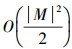2)对于至少含有两个顶点属性的情况，本文计算每个概念的算法复杂度O(|M|2)。

3)李立峰等仅是从理论方面指出弦二部图的概念格表示，并没有给出算法过程。所以他们的方法只是理论过程，而无法直接实现。

3 实例

 对像 a1 a2 a3 a4 a5 a6 a7 1 × × × 2 × × × 3 × × × 4 × × × 5 × × × 6 × × 7 × × 8 × × × 9 × × 10 × ×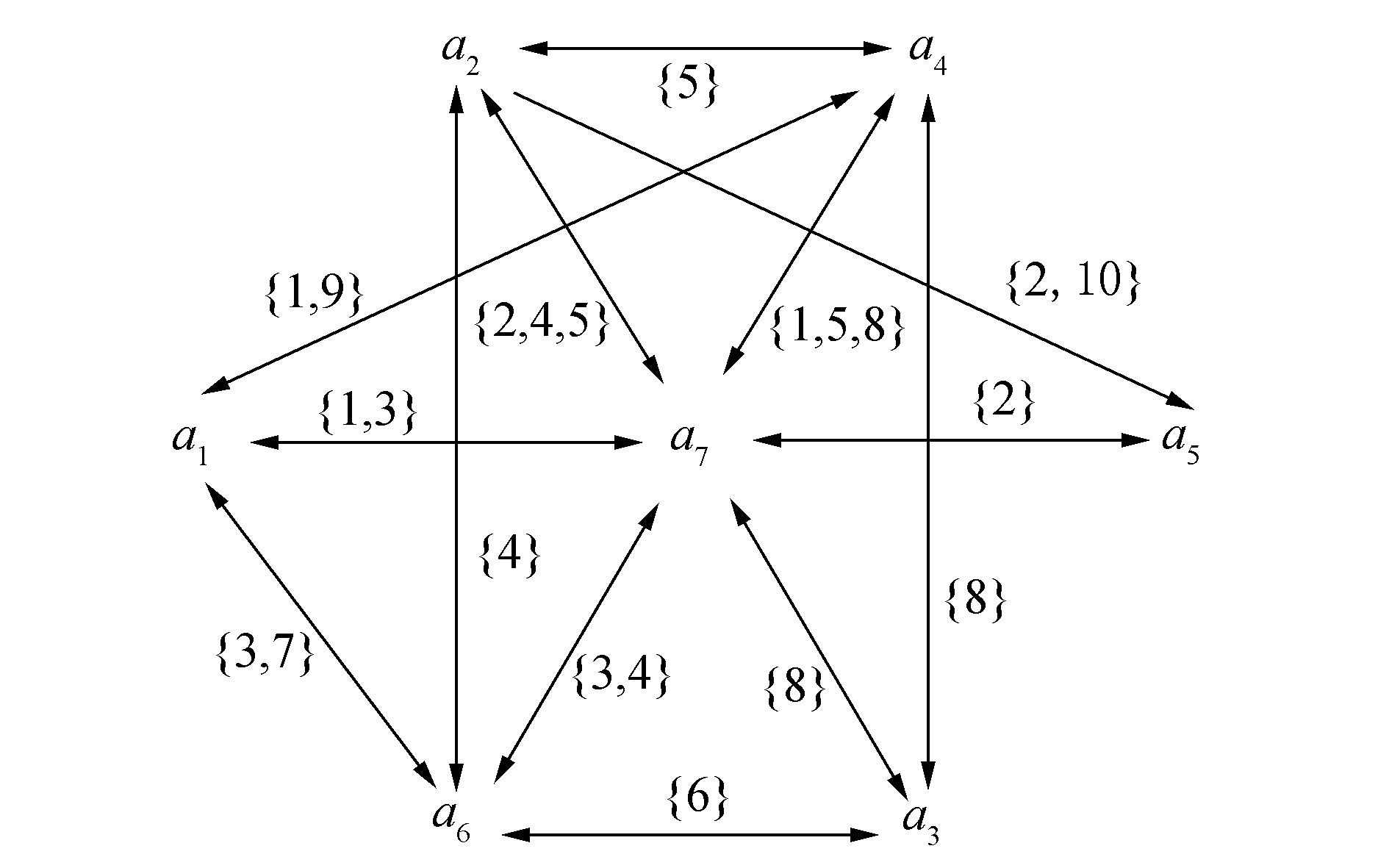图 4 (A(O1,M1,I1)，w) Fig. 4 (A(O1,M1,I1)，w)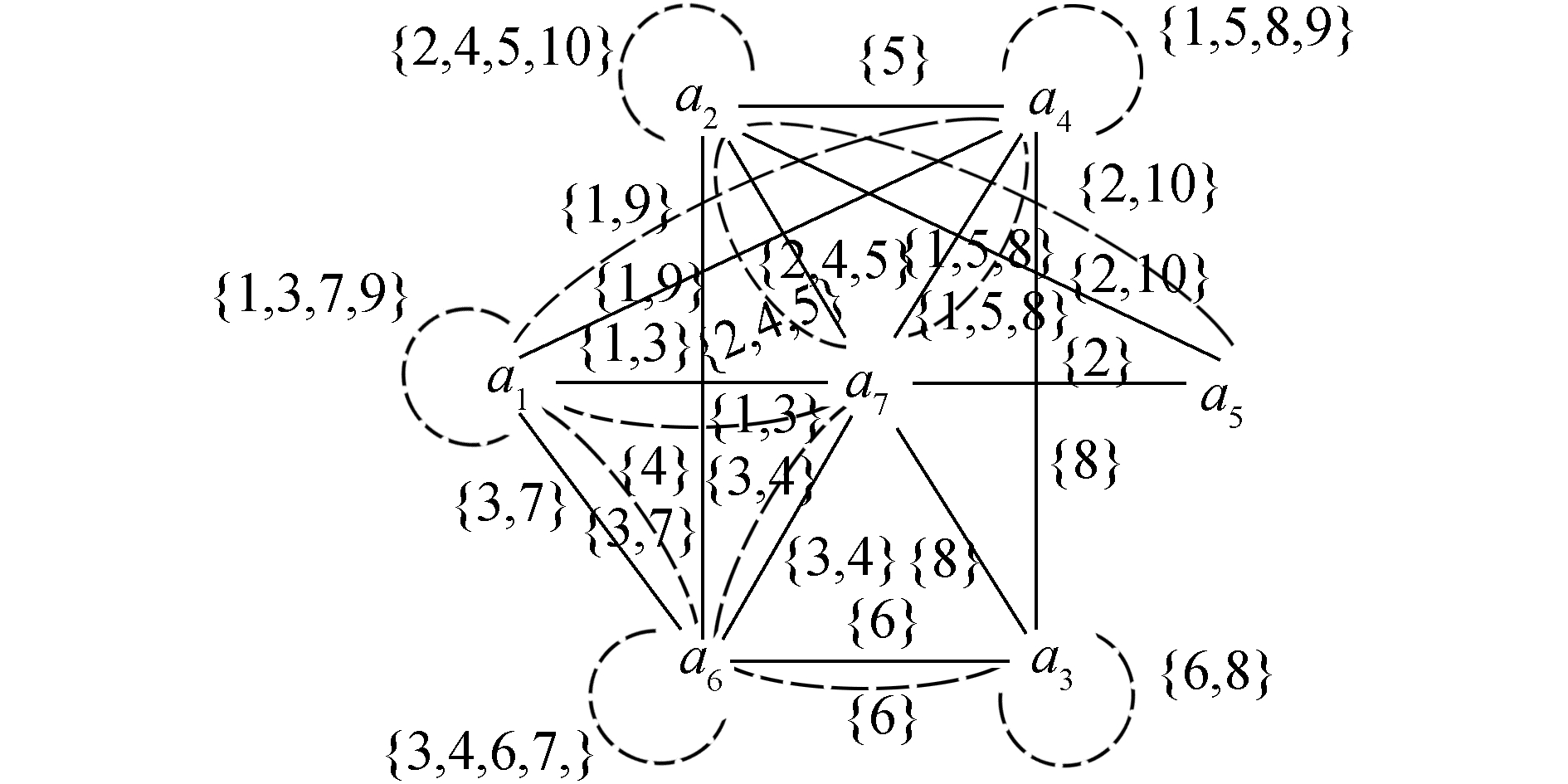图 5 (R(O1,M1,I1)，w) Fig. 5 (R(O1,M1,I1)，w)

3)对任意两个元素wi，wjWi，j=1,2,…,16,取交集，此过程需要进行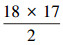次，得到W∪{1}∪{3}，对{{1}，{3}}执行步骤3)中②，此过程进行1次，可以看出符合3)中①，可转4)。

4)取W中的元素w(a7,a7)，判断w(a7,a7)⊆w(ai,aj) 其中i，j=1,2,…,20，每个元素比较20次，寻找最大圈，得到概念(1 2 3 4 5 8，a7)。

5)依次取W中的其他元素，重复4)，在此例W中的18个元素，4)需要重复18次。

4 结束语

  WILLE R. Restructuring lattice theory:an approach based on hierarchies of concepts[M]//RIVAL I. Ordered Sets. Dordrecht:Springer, 1982.  BELOHLAVEK R, SIGMUND E, ZACPAL J. Evaluation of IPAQ questionnaires supported by formal concept analysis[J]. Information sciences , 2011, 181 (10) : 1774-1786 DOI:10.1016/j.ins.2010.04.011  NGUYEN T T, HUI S C, CHANG Kuiyu. A lattice-based approach for mathematical search using formal concept analysis[J]. Expert systems with applications , 2012, 39 (5) : 5820-5828 DOI:10.1016/j.eswa.2011.11.085  王旭杨, 李明. 基于概念格的数据挖掘方法研究[J]. 计算机应用 , 2005, 25 (4) : 827-829 WANG Xuyang, LI Ming. Method of data mining based on concept lattice[J]. Computer applications , 2005, 25 (4) : 827-829  SIFF M, REPS T. Identifying modules via concept analysis[C]//Proceedings of International Conference on Software Maintenance. Washington, DC, USA:IEEE Computer Society, 1997:170-179.  FERJANI F, ELLOUMI S, JAOUA A, et al. Formal context coverage based on isolated labels:an efficient solution for text feature extraction[J]. Information sciences , 2012, 188 : 198-214 DOI:10.1016/j.ins.2011.10.023  邓君, 马晓君, 张巨峰, 等. 基于概念格的实体档案馆用户行为研究[J]. 图书情报工作 , 2014, 58 (12) : 109-117 DENG Jun, MA Xiaojun, ZHANG Jufeng, et al. Study on entity archives' user behavior based on concept lattice[J]. Library and information service , 2014, 58 (12) : 109-117  张晓, 龙伟, 卢斌. 基于概念格的关联规则在排产管理的应用[J]. 计算机工程与应用 , 2014, 50 (9) : 264-270 v ZHANG Xiao, LONG Wei, LU Bin. Application of association rule based on concept lattice for scheduling management[J]. Computer engineering and applications , 2014, 50 (9) : 264-270  张涛, 任宏雷. 形式背景的属性拓扑表示[J]. 小型微型计算机系统 , 2014, 35 (3) : 590-593 ZHANG Tao, REN Honglei. Attribute topology of formal context[J]. Journal of Chinese computer systems , 2014, 35 (3) : 590-593  BERRY A, SIGAYRET A. Representing a concept lattice by a graph[J]. Discrete applied mathematics , 2004, 144 (1/2) : 27-42  李立峰, 刘三阳, 罗清君. 弦二部图的概念格表示[J]. 电子学报 , 2013, 41 (7) : 1384-1388 LI Lifeng, LIU Sanyang, LUO Qingjun. Representing chordal bipartite graph using concept lattice theory[J]. Acta electronica sinica , 2013, 41 (7) : 1384-1388  DAVEY B A, PRIESTLEY H A. Introduction to lattices and order[M]. 2nd ed New York: Cambridge University Press, 2002 : 66 -69.  王树禾. 图论[M]. 北京: 科学出版社, 2009 .  ZHANG Tao, REN Honglei, WANG Xiaomin. A calculation of formal concept by attribute topology[J]. ICIC express letters part B:applications , 2013, 4 (3) : 793-800  方嘉琳. 集合论[M]. 长春: 吉林人民出版社, 1982 .
DOI: 10.11992/tis.201606006

0

#### 文章信息

MAO Hua, LIU Yichao

An algorithm for concept lattice construction based on maximum cycles of weight values

CAAI Transactions on Intelligent Systems, 2016, 11(4): 519-525
http://dx.doi.org/10.11992/tis.201606006